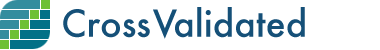## Scores for Questions and Answers (compared by Month)

0

(click on this box to dismiss)Q&A for people interested in statistics, machine learning, data analysis, data mining, and data visualization

``````select [Month],
case when (cast(sum(case when PostTypeId = 2 then 1 else 0 end) as float)>0)
then  log(cast(cast(sum(case when PostTypeId = 2 then Score else 0 end) as float )/
cast(sum(case when PostTypeId = 2 then 1 else 0 end) as float)
as Decimal(6,3)),10)
case when (cast(sum(case when PostTypeId = 1 then 1 else 0 end) as float)>0)
then  log(cast(cast(sum(case when PostTypeId = 1 then Score else 0 end) as float )/
cast(sum(case when PostTypeId = 1 then 1 else 0 end) as float)
as Decimal(6,3)),10)
else (null) end ScoreQuestions
from
(
select CAST(
cast(DATEPART(YYYY, CreationDate) as varchar) + '-' + cast(DATEPART(MM, CreationDate) as varchar) + '-01'
as datetime) [Month],
PostTypeId,
Score
from Posts p
) as X
group by [Month]
order by [Month] asc``````

### Enter Parameters

Options:
-Hold tight while we fetch your results
:records returned in :time ms:cached